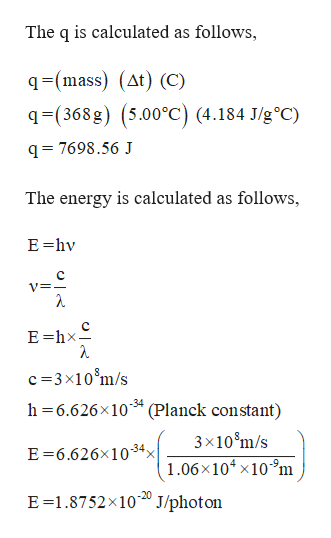# 12. A 368 g sample of water absorbs infrared radiation at 1.06 × 104 nm from a carbon dioxide laser. Suppose all the absorbed radiation is converted to heat. Calculate the number of photons at this wavelength required to raise the temperature of the water by 5.00ºC.

Question
456 views

12. A 368 g sample of water absorbs infrared radiation at 1.06 × 104 nm from a carbon dioxide laser. Suppose all the absorbed radiation is converted to heat. Calculate the number of photons at this wavelength required to raise the temperature of the water by 5.00ºC.

check_circle

Step 1

Mass of water = 368g

Specific heat of water = 4.184 J/go ...help_outlineImage TranscriptioncloseThe q is calculated as follows, q-(mass) (At) (C) q-(368g) (5.00°C) (4.184 J/g°C) q= 7698.56 J The energy is calculated as follows, E hv C V= E hx c 3x10 m/s h 6.626x10 (Planck constant) -34 3x10 m/s E 6.626x10-34, 1.06x104 x109m E 1.8752x1020 J/photon fullscreen

### Want to see the full answer?

See Solution

#### Want to see this answer and more?

Solutions are written by subject experts who are available 24/7. Questions are typically answered within 1 hour.*

See Solution
*Response times may vary by subject and question.
Tagged in

### General Chemistry